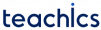# Hashing

In this tutorial, you will learn what is hashing, what a hash table is and what is a hash function.

Hashing is a technique of mapping a large set of data into tabular indices.

### The importance of Hashing

We know that the time complexity of search algorithms depends upon the number of elements in the list. Linear search and binary search algorithms have a time complexity of `O(n)` and `O(log n) `respectively. Hashing is a search technique which is independent of the number of elements in the list. It uniquely identify a specific item from a group of similar items. Therefore, hashing allows searching, updating and retrieval of data in a constant time, that is `O(1)`.

### Hash table

A hash table is a data structure that stores data as key-value pairs. Each key is matched to a value in the hash table.

• Keys are used to index the data.
• Value specifies the data associated with the keys.

## Hash Function

A hash function is a mathematical formula, used for mapping keys into table indices. This process of mapping the keys to corresponding indices in a hash table is called hashing.

Assume `k` is a key and `h(x)` is a hash function. In a hash table, an element linked with key `k` will be stored at the index `h(k)`.

The following figure shows a hash table in which each key from the set K is mapped to indices generated by a hash function.

#### Characteristics of good hash function

• It should be very simple and quick to compute.
• The cost of execution must be low.
• It should distribute the hash addresses as evenly as possible within L, so there are fewer collisions.
• The same hash value must be generated for a given input value.

Lets have a look at dIfferent hash functions which use numeric keys.

#### Division Method

Choose a number m larger than the number n of keys in K. m is normally chosen to be a prime number. This method divides x by M and then uses the reminder obtained. The hash function is given by

`H(k) = k mod m`

For example: Suppose K = 1234, setting M=97, hash values can be calculated as:

`h(1234) = 1234 % 97 = 70 `

#### Multiplication Method

The following steps are involved in multiplication method.

1. Choose a constant A such that 0 < A < 1
2. Multiply k by A
3. Extract the fractional part
4. Multiply the result by the size of hash table (m).

The hash function can be given as:

`h(k) = ⌊ m (kA mod 1) ⌋`

The best choice for A is 0.6180339887.

For example: Suppose k = 12345 and the size of the table is 1000. The hash value can be calculated as:

```h(12345) = ⌊ 1000 (12345 * 0.618033 mod 1) ⌋
‎         = ⌊ 1000 (7629.617385 mod 1) ⌋
‎         = ⌊ 1000 (0.617385) ⌋
‎         = ⌊ 617.385 ⌋
‎         = 617```

#### Mid-Square Method

The mid-square method works in the following steps:

1. Find square of the key.
2. Extract the middle r digits of the result.

The hash function can be given by:

`h(k) = s`

where s is obtained by selecting r digits of k2.

For example: Suppose k = 1234. Then let’s find the hash value for a hash table of size 100.

Since the index of hash table varies between 0 and 99, we can choose `r = 2` . Then:

k = 1234, k2 = 1522756, h(1234) = 27

#### Folding Method

• The key k is divided into a number of parts of same length k1, k2, … , kr.
• And they are added together ignoring last carry if any.

The hash function can be given by:

h(k) = k1 + k2 + … + kr

Given a hash table of 100 locations, calculate the hash value using folding method for keys 5678.

key 6789
Parts 67 and 89
Sum 156
Hash Value 34

## Hash Collision and Collision Resolution

When hash function maps two different keys to the same location, collision is occurred. Since we cannot store two records in the same location we solve the problem by using collision resolution techniques. Two most popular collision resolution techniques are: